# Chemistry Topic-wise Test - 3

## 45 Questions MCQ Test NEET Mock Test Series & Past Year Papers | Chemistry Topic-wise Test - 3

Description
This mock test of Chemistry Topic-wise Test - 3 for NEET helps you for every NEET entrance exam. This contains 45 Multiple Choice Questions for NEET Chemistry Topic-wise Test - 3 (mcq) to study with solutions a complete question bank. The solved questions answers in this Chemistry Topic-wise Test - 3 quiz give you a good mix of easy questions and tough questions. NEET students definitely take this Chemistry Topic-wise Test - 3 exercise for a better result in the exam. You can find other Chemistry Topic-wise Test - 3 extra questions, long questions & short questions for NEET on EduRev as well by searching above.
QUESTION: 1

Solution:
QUESTION: 2

Solution:
QUESTION: 3

### The nature of inter-molecular forces among benzene molecules is

Solution:
QUESTION: 4
The absolute configuration of the following compound is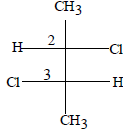Solution:
QUESTION: 5
Correct order of stability is
Solution:
QUESTION: 6
The addition of HCN to a carbonyl compound is an example of
Solution:
QUESTION: 7
Acetaldehyde is the rearrangement product of
Solution:
QUESTION: 8
The replacement of chlorine of chlorobenzene to give phenol requires drastic conditions, but the chlorine of 2,4-dinitrochlorobenzene is readily replaced since
Solution:
QUESTION: 9
The boiling point of neopentane will be more than that of
Solution:
QUESTION: 10
In the presence of platinum catalyst, hydrocarbon A adds hydrogen to form n-hexane. When hydrogen bromide is added to A instead of hydrogen, only a single bromo compound is formed. Which of the following is A ?
Solution:
QUESTION: 11
Which of the following will convert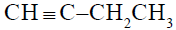to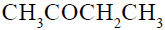?
Solution:
QUESTION: 12
1,3-Butadiene when treated with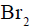gives
Solution:
QUESTION: 13
Ozonolysis of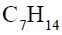gave 2-methyl-3-pentanone. The alkene is
Solution: A) is right ans bcoz in above given option only a give 2me 3pantanone .in ozonolysis compound break at double bond.
QUESTION: 14
A gas on passing through ammonical solution of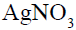does not give any precipitate but decolourises alkaline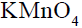solution. The gas may be
Solution:
QUESTION: 15
The ortho-para directing group among the following is
Solution:
QUESTION: 16
Amongst the following, the compound that can be most readily sulphonated is
Solution:
QUESTION: 17
Which of the chemical system is non-aromatic ?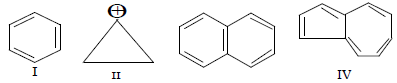Solution:
QUESTION: 18
In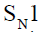(substitution, nucleophilic unimoleuclar) a reaction, the racemization takes place. It is due to
Solution:
QUESTION: 19
Chloroform on warming with Ag powder gives
Solution:
QUESTION: 20
Ethyl alcohol gives ethyl chloride with the help of
Solution:
QUESTION: 21
Which of the following compound will make precipitate most readily with?
Solution:
QUESTION: 22
Which of the following is responsible for depletion of the ozone layer in the upper strata of the atmosphere?
Solution:
QUESTION: 23
How much of NaOH is required to neutralise 1500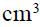of 0.1 N HCl ? (Na = 23)
Solution:
QUESTION: 24
The number of water molecules present in a drop of water (volume 0.0018 ml) at room temperature is
Solution:
QUESTION: 25
The correct representation of Charle’s law is given by
Solution:
QUESTION: 26
At high pressure, the compressibility factor ‘Z’ is equal to
Solution:
QUESTION: 27
The ratio of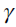for inert gases is
Solution:
QUESTION: 28
The structure of TlCl is similar to CsCl. What would be the radius ratio in TlCl ?
Solution:
QUESTION: 29
In the sphalerite (ZnS) structure,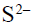ions form a face- centred cubic lattic. Then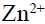ions are present on the body diagonals at
Solution:
QUESTION: 30
Which substance shows antiferromagnetism ?
Solution:
QUESTION: 31
Line spectra is characteristic of
Solution:
QUESTION: 32
The orbital angular momentum of an electron in 2 s orbitals is
Solution:
QUESTION: 33
Which of the following molecule is linear ?
Solution:
QUESTION: 34
The correct order of hybridization of the central atom in the following species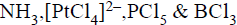is
Solution:
QUESTION: 35
A diatomic molecule has a dipole moment of 1.2 D. If the bond distance is 1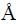, what percentage of electronic charge exists on each atom ?
Solution:
QUESTION: 36
Enthalpy of a reaction at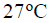is 15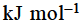. The reaction will be feasible if entropy is
Solution:
QUESTION: 37
Heat of neutralisation is least when
Solution:
QUESTION: 38
Starting with one mole of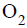and two moles of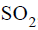the equilibrium for the formation of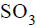was established at a certain temperature. If V is the volume of the vessel and 2x is the number of moles ofpresent, the equilibrium constant will be
Solution:
QUESTION: 39
For equilibrium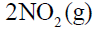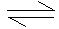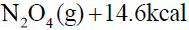increase of temperature would
Solution:
QUESTION: 40
Ionic product of water is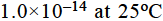. This is so
Solution:
QUESTION: 41
The precipitate of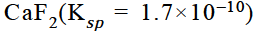is obtained on mixing equal volumes of
Solution:
QUESTION: 42
A hydride of nitrogen which as acidic is
Solution:
QUESTION: 43
Which one of the following removes temporary hardness of water ?
Solution:
QUESTION: 44
NaH has ....... types of structure
Solution:
QUESTION: 45
Which one of the following is least soluble in water
Solution: Mg have 2 electrons in its valence shell. And it has given these electrons to F atoms.